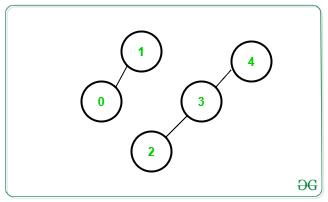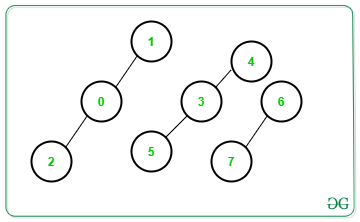Related Articles

# Number of connected components of a graph ( using Disjoint Set Union )

• Difficulty Level : Hard
• Last Updated : 25 Aug, 2021

Given an undirected graph G with vertices numbered in the range [0, N] and an array Edges[][] consisting of M edges, the task is to find the total number of connected components in the graph using Disjoint Set Union algorithm.

Examples:

Input: N = 4, Edges[][] = {{1, 0}, {2, 3}, {3, 4}}
Output: 2
Explanation: There are only 2 connected components as shown below:Input: N = 4, Edges[][] = {{1, 0}, {0, 2}, {3, 5}, {3, 4}, {6, 7}}
Output: 3
Explanation: There are only 3 connected components as shown below:Approach: The problem can be solved using Disjoint Set Union algorithm. Follow the steps below to solve the problem:

• In DSU algorithm, there are two main functions, i.e. connect() and root() function.
• connect(): Connects an edge.
• root(): Recursively determine the topmost parent of a given edge.
• For each edge {a, b}, check if a is connected to b or not. If found to be false, connect them by appending their top parents.
• After completing the above step for every edge, print the total number of the distinct top-most parents for each vertex.

Below is the implementation of the above approach:

## C++

 `// C++ program for the above approach` `#include ``using` `namespace` `std;` `// Stores the parent of each vertex``int` `parent;` `// Function to find the topmost``// parent of vertex a``int` `root(``int` `a)``{``    ``// If current vertex is``    ``// the topmost vertex``    ``if` `(a == parent[a]) {``        ``return` `a;``    ``}` `    ``// Otherwise, set topmost vertex of``    ``// its parent as its topmost vertex``    ``return` `parent[a] = root(parent[a]);``}` `// Function to connect the component``// having vertex a with the component``// having vertex b``void` `connect(``int` `a, ``int` `b)``{``    ``// Connect edges``    ``a = root(a);``    ``b = root(b);` `    ``if` `(a != b) {``        ``parent[b] = a;``    ``}``}` `// Function to find unique top most parents``void` `connectedComponents(``int` `n)``{``    ``set<``int``> s;` `    ``// Traverse all vertices``    ``for` `(``int` `i = 0; i < n; i++) {` `        ``// Insert all topmost``        ``// vertices obtained``        ``s.insert(root(parent[i]));``    ``}` `    ``// Print count of connected components``    ``cout << s.size() << ``'\n'``;``}` `// Function to print answer``void` `printAnswer(``int` `N,``                 ``vector > edges)``{` `    ``// Setting parent to itself``    ``for` `(``int` `i = 0; i <= N; i++) {``        ``parent[i] = i;``    ``}` `    ``// Traverse all edges``    ``for` `(``int` `i = 0; i < edges.size(); i++) {``        ``connect(edges[i], edges[i]);``    ``}` `    ``// Print answer``    ``connectedComponents(N);``}` `// Driver Code``int` `main()``{``    ``// Given N``    ``int` `N = 8;` `    ``// Given edges``    ``vector > edges = {``        ``{ 1, 0 }, { 0, 2 }, { 5, 3 }, { 3, 4 }, { 6, 7 }``    ``};` `    ``// Function call``    ``printAnswer(N, edges);` `    ``return` `0;``}`

## Java

 `// Java program for the above approach``import` `java.util.*;``class` `GFG{` `// Stores the parent of each vertex``static` `int` `[]parent = ``new` `int``[``1000000``];` `// Function to find the topmost``// parent of vertex a``static` `int` `root(``int` `a)``{``  ` `    ``// If current vertex is``    ``// the topmost vertex``    ``if` `(a == parent[a])``    ``{``        ``return` `a;``    ``}` `    ``// Otherwise, set topmost vertex of``    ``// its parent as its topmost vertex``    ``return` `parent[a] = root(parent[a]);``}` `// Function to connect the component``// having vertex a with the component``// having vertex b``static` `void` `connect(``int` `a, ``int` `b)``{``  ` `    ``// Connect edges``    ``a = root(a);``    ``b = root(b);` `    ``if` `(a != b) {``        ``parent[b] = a;``    ``}``}` `// Function to find unique top most parents``static` `void` `connectedComponents(``int` `n)``{``    ``HashSet s = ``new` `HashSet();` `    ``// Traverse all vertices``    ``for` `(``int` `i = ``0``; i < n; i++)``    ``{` `        ``// Insert all topmost``        ``// vertices obtained``        ``s.add(parent[i]);``    ``}` `    ``// Print count of connected components``    ``System.out.println(s.size());``}` `// Function to print answer``static` `void` `printAnswer(``int` `N,``int` `[][] edges)``{` `    ``// Setting parent to itself``    ``for` `(``int` `i = ``0``; i <= N; i++)``    ``{``        ``parent[i] = i;``    ``}` `    ``// Traverse all edges``    ``for` `(``int` `i = ``0``; i < edges.length; i++)``    ``{``        ``connect(edges[i][``0``], edges[i][``1``]);``    ``}` `    ``// Print answer``    ``connectedComponents(N);``}` `// Driver Code``public` `static` `void` `main(String[] args)``{``  ` `    ``// Given N``    ``int` `N = ``8``;` `    ``// Given edges``   ``int` `[][]edges = {{ ``1``, ``0` `}, { ``0``, ``2` `},``                    ``{ ``5``, ``3` `}, { ``3``, ``4` `},``                    ``{ ``6``, ``7` `}};` `    ``// Function call``    ``printAnswer(N, edges);``}``}` `// This code is contributed by 29AjayKumar`

## Python3

 `# Python3 program for the above approach` `# Stores the parent of each vertex``parent ``=` `[``0``]``*``(``1000000``)`` ` `# Function to find the topmost``# parent of vertex a``def` `root(a) :``   ` `    ``# If current vertex is``    ``# the topmost vertex``    ``if` `(a ``=``=` `parent[a]) :       ``        ``return` `a``        ` `    ``# Otherwise, set topmost vertex of``    ``# its parent as its topmost vertex``    ``parent[a] ``=` `root(parent[a])``    ``return` `parent[a]``    ` `# Function to connect the component``# having vertex a with the component``# having vertex b``def` `connect(a, b) :``   ` `    ``# Connect edges``    ``a ``=` `root(a)``    ``b ``=` `root(b)`` ` `    ``if` `(a !``=` `b) :``        ``parent[b] ``=` `a``        ` `# Function to find unique top most parents``def` `connectedComponents(n) :` `    ``s ``=` `set``()`` ` `    ``# Traverse all vertices``    ``for` `i ``in` `range``(n) :`` ` `        ``# Insert all topmost``        ``# vertices obtained``        ``s.add(root(parent[i]))`` ` `    ``# Print count of connected components``    ``print``(``len``(s))``    ` `# Function to print answer``def` `printAnswer(N, edges) :`` ` `    ``# Setting parent to itself``    ``for` `i ``in` `range``(N ``+` `1``) :  ``        ``parent[i] ``=` `i`` ` `    ``# Traverse all edges``    ``for` `i ``in` `range``(``len``(edges)) :   ``        ``connect(edges[i][``0``], edges[i][``1``])`` ` `    ``# Print answer``    ``connectedComponents(N)``    ` `# Given N``N ``=` `6` `# Given edges``edges ``=` `[[ ``1``, ``0` `], [ ``2``, ``3` `], [ ``1``, ``2` `], [ ``4``, ``5` `]]` `# Function call``printAnswer(N, edges)` `# This code is contributed by divyesh072019`

## C#

 `// C# program for the above approach``using` `System;``using` `System.Collections.Generic;``class` `GFG {``    ` `    ``// Stores the parent of each vertex``    ``static` `int``[] parent = ``new` `int``;``      ` `    ``// Function to find the topmost``    ``// parent of vertex a``    ``static` `int` `root(``int` `a)``    ``{``        ``// If current vertex is``        ``// the topmost vertex``        ``if` `(a == parent[a]) {``            ``return` `a;``        ``}``      ` `        ``// Otherwise, set topmost vertex of``        ``// its parent as its topmost vertex``        ``return` `parent[a] = root(parent[a]);``    ``}``      ` `    ``// Function to connect the component``    ``// having vertex a with the component``    ``// having vertex b``    ``static` `void` `connect(``int` `a, ``int` `b)``    ``{``        ``// Connect edges``        ``a = root(a);``        ``b = root(b);``      ` `        ``if` `(a != b) {``            ``parent[b] = a;``        ``}``    ``}``      ` `    ``// Function to find unique top most parents``    ``static` `void` `connectedComponents(``int` `n)``    ``{``        ``HashSet<``int``> s = ``new` `HashSet<``int``>();``      ` `        ``// Traverse all vertices``        ``for` `(``int` `i = 0; i < n; i++) {``      ` `            ``// Insert all topmost``            ``// vertices obtained``            ``s.Add(parent[i]);``        ``}``      ` `        ``// Print count of connected components``        ``Console.WriteLine(s.Count);``    ``}``      ` `    ``// Function to print answer``    ``static` `void` `printAnswer(``int` `N, List > edges)``    ``{``      ` `        ``// Setting parent to itself``        ``for` `(``int` `i = 0; i <= N; i++) {``            ``parent[i] = i;``        ``}``      ` `        ``// Traverse all edges``        ``for` `(``int` `i = 0; i < edges.Count; i++) {``            ``connect(edges[i], edges[i]);``        ``}``      ` `        ``// Print answer``        ``connectedComponents(N);``    ``}  ` `  ``// Driver code``  ``static` `void` `Main() {``      ` `    ``// Given N``    ``int` `N = 8;``  ` `    ``// Given edges``    ``List> edges = ``new` `List>();``    ``edges.Add(``new` `List<``int``> { 1, 0 });``    ``edges.Add(``new` `List<``int``> { 0, 2 });``    ``edges.Add(``new` `List<``int``> { 5, 3 });``    ``edges.Add(``new` `List<``int``> { 3, 4 });``    ``edges.Add(``new` `List<``int``> { 6, 7 });``  ` `    ``// Function call``    ``printAnswer(N, edges);``  ``}``}` `// This code is contributed by divyeshrabadiya07`

## Javascript

 ``
Output:
`3`

Time Complexity: O(N+M)
Auxiliary Space: O(N+M)

Attention reader! Don’t stop learning now. Get hold of all the important DSA concepts with the DSA Self Paced Course at a student-friendly price and become industry ready.  To complete your preparation from learning a language to DS Algo and many more,  please refer Complete Interview Preparation Course.

In case you wish to attend live classes with experts, please refer DSA Live Classes for Working Professionals and Competitive Programming Live for Students.

My Personal Notes arrow_drop_up